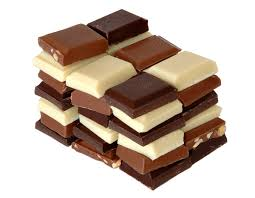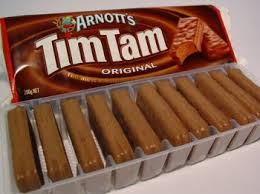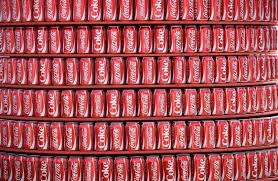## Tuesday, 8 September 2015

### Math problemsAmon has 6 packets of chocolate.
There are 24 chocolates in each packet.
How many does Amon have?
142 because
split 24 into 12 and 12 then 6x12=72
72+72=142Viliami has 4 packets of tim tams.
There are 28 tim tams in each packet.
How many does Viliami have?
112 because
split 28 into 14 and 14 then 4x14=56
then 56+56=112Soane has 6 packets of cans.
there are 16 cans in each packet
How many does Soane have?
96 because
split the 16 into 10 and 6 then
6x10=60 and then 6x6=36
then 60+36=96